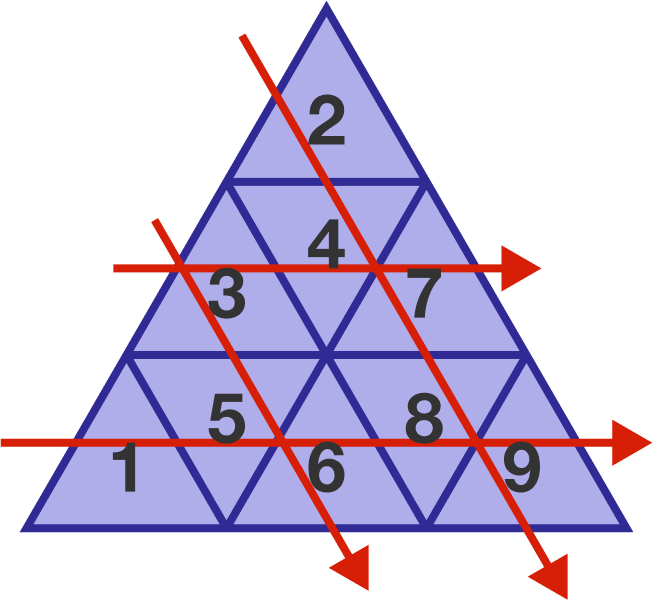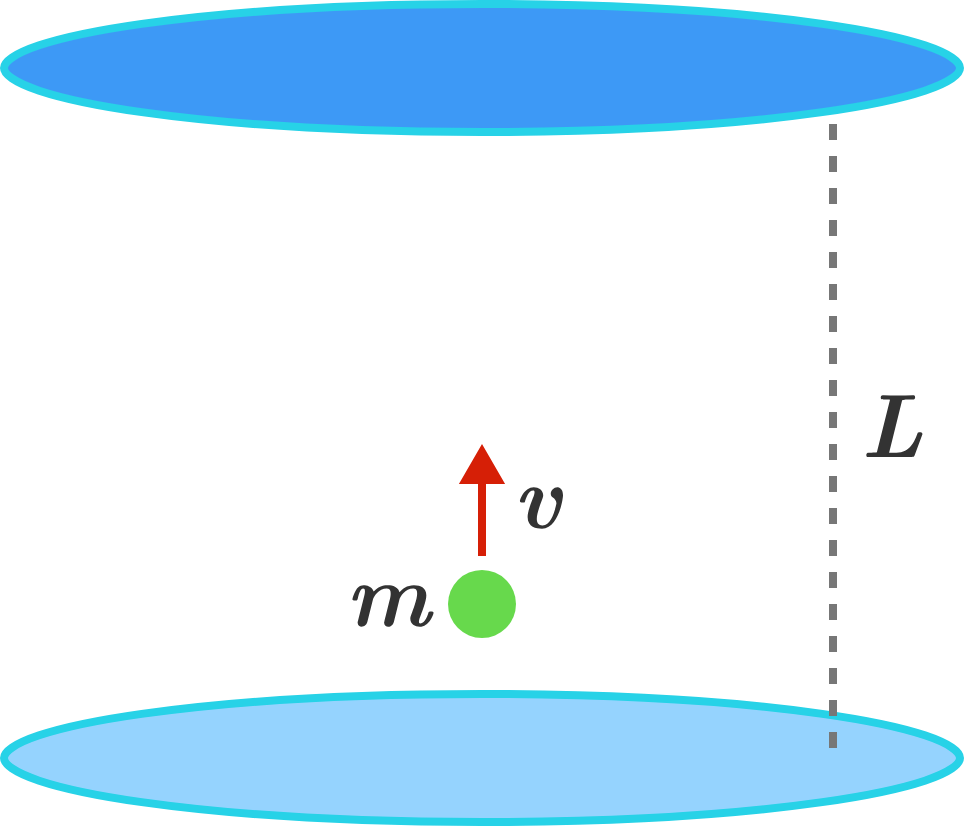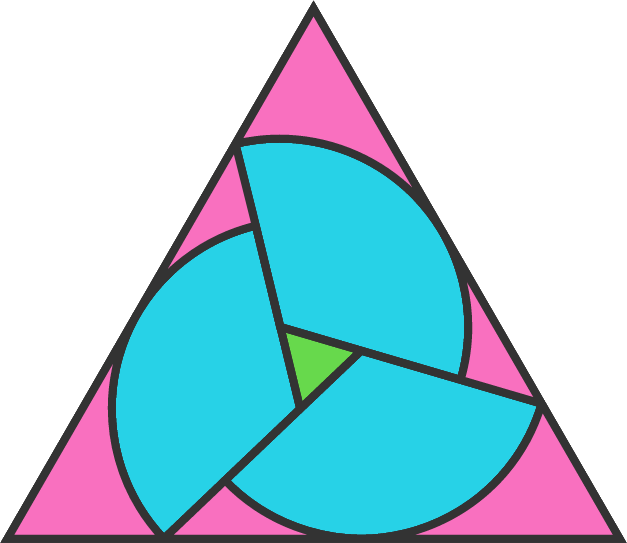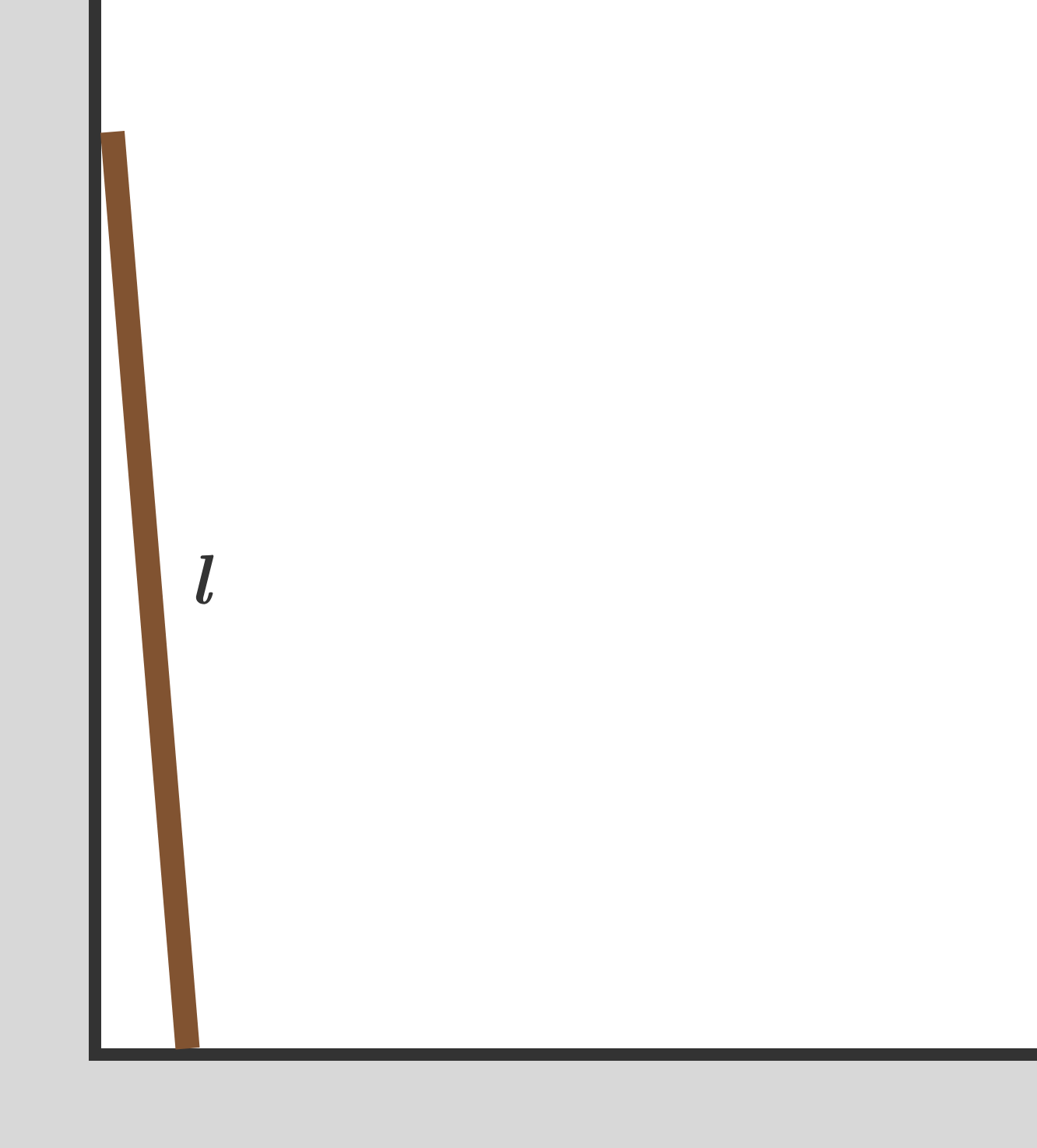# Problems of the Week

Contribute a problem

How many ways can you put all the numbers 1 through 9 in this triangular grid, such that numbers along each arrow form an increasing sequence?The diagram shows one possible configuration.A ball of mass $m$ is moving between two parallel plates of mass much larger than $m$, separated by distance $L$. The ball moves with velocity $v$ in the direction perpendicular to the plates and collides with the plates multiple times. Due to the momentum transfer at the collisions, each plate experiences an average force of $F$ that is acting to push the plates apart.

Initially, the velocity of the ball is $v_0$ and the distance between the plates is $L_0$. As the separation of the planes is slowly changed, the force is changing and it can be expressed as $F= \frac{m v_0^2}{ L} \left(\frac{L_0}{L}\right)^A.$ What is the value of $A?$

Assume that the collision between the ball and the plates is perfectly elastic. Neglect the effect of gravity.A circle of radius $20$ is sliced into three congruent sectors, which are then slid apart to create a green equilateral triangle with side length $9.$ A larger equilateral triangle is then circumscribed.

The side length of this large triangle can be written as $\frac{a+b\sqrt{d}}{c}$ with $d$ square-free and $a, b, c$ irreducible.

What is the value of $a+b+c+d?$

A line is "special" to a curve if it is both a tangent and a normal of the curve.

Only two lines are special to the curve $x^3+ax^2+bx+c,$ where $a,b,c$ are constants, and the two lines have the same slope.

What is this slope?An $8.1\text{ m}$-long uniform ladder stands on a frictionless floor and leans against a frictionless wall. It is initially held motionless, with its bottom end at a very small distance from the wall.

The ladder is then released from rest.

What is the speed of the ladder's tip when it hits the ground?

Note: Take $g=10\text{ m}/\text{s}^2.$

×